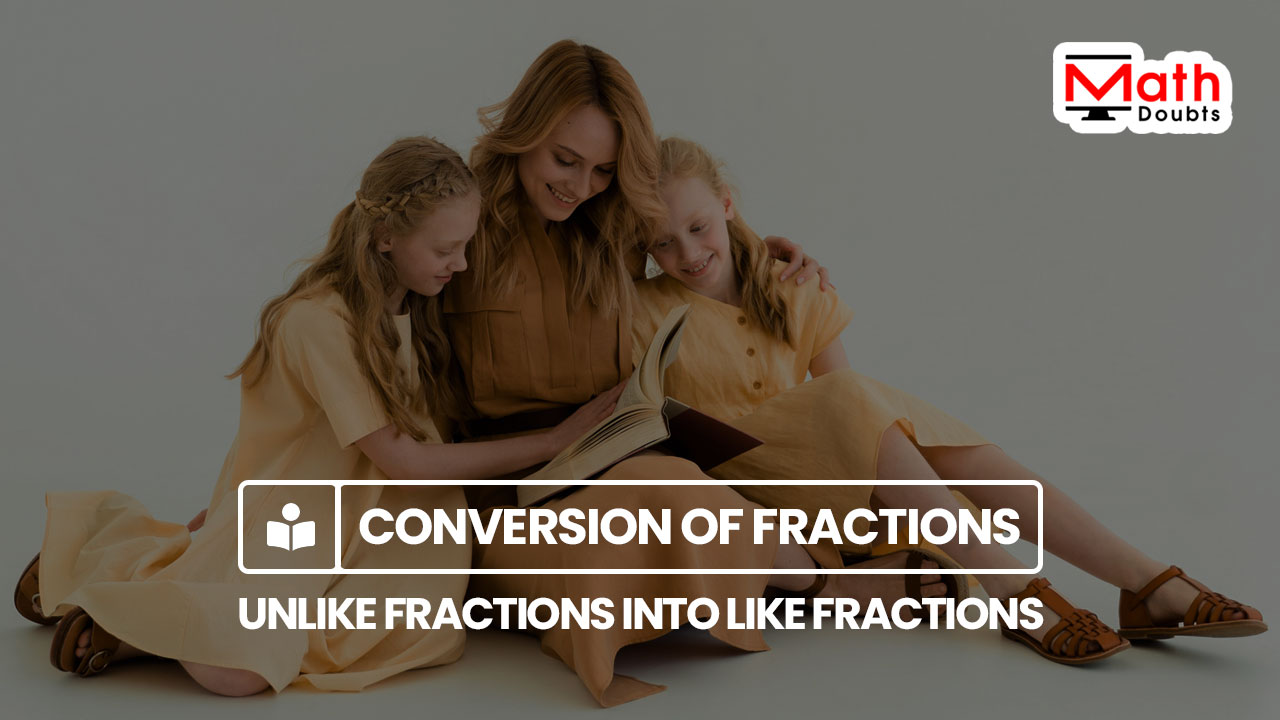# How to Convert the fractions with different denominators into the fractions with same denominator

## Definition

A mathematical process of converting the fractions with different denominators into the fractions with the fractions with same denominator is called the conversion of the unlike fractions as the like fractions.

### Introduction

The fractions with different denominators create problems to us in some mathematical operations but everything will be smoothly done in the case of the fractions with same denominator. So, converting the fractions with different denominators into the fractions with same denominator helps us to do every mathematical operation easily.There are three simple steps to convert two or more unlike fractions into like fractions.

1. Find the least common multiple (L.C.M) of the denominators of the given fractions.
2. Divide the lowest common multiple (L.C.M) by each denominator to obtain their quotient.
3. Finally, multiply both numerator and denominator of the every fraction by the respective quotient.

Now, let’s learn how to use these steps to convert the fractions with different denominators into the fractions with the same denominator from an example.

#### Example

$\dfrac{1}{2},$ $\dfrac{5}{6}$ and $\dfrac{8}{9}$

Look at the denominators of the given fractions. The denominators are different and they are $2,$ $6$ and $9$, which express that the given fractions are unlike fractions.

Firstly, find the least common multiple (L.C.M) of the denominators $2,$ $6$ and $9$. It is evaluated that the LCM of the denominators $2,$ $6$ and $9$ is equal to $18$.

Now, divide the smallest common multiple (L.C.M) $18$ by each denominator to find their quotient.

$(1).\,\,$ $\dfrac{18}{2}$ $\,=\,$ $9$

The quotient of $18$ divided by $2$ is equal to $9$.

$(2).\,\,$ $\dfrac{18}{6}$ $\,=\,$ $3$

The quotient of $18$ divided by $6$ is equal to $3$.

$(3).\,\,$ $\dfrac{18}{9}$ $\,=\,$ $2$

The quotient of $18$ divided by $9$ is equal to $2$.

Finally, multiply the numerator and denominator of each unlike fraction by the respective quotient to complete the process of transforming the unlike fractions as the like fractions.

$(1).\,\,$ $\dfrac{9 \times 1}{9 \times 2}$ $\,=\,$ $\dfrac{9}{18}$

$(2).\,\,$ $\dfrac{3 \times 5}{3 \times 6}$ $\,=\,$ $\dfrac{15}{18}$

$(3).\,\,$ $\dfrac{2 \times 8}{2 \times 9}$ $\,=\,$ $\dfrac{16}{18}$

The fractions with different denominators $\dfrac{1}{2},$ $\dfrac{5}{6}$ and $\dfrac{8}{9}$ are successfully converted as the fractions with same denominator $\dfrac{9}{18},$ $\dfrac{15}{18}$ and $\dfrac{16}{18}$ respectively.

In this way, any two or more unlike fractions can be converted as the like fractions in mathematics.

#### Worksheet

The list of questions on converting the unlike fractions into the like fractions for your practice and examples with solutions to learn how to convert the fractions with different denominators as the fractions with same denominator.

Latest Math Topics
Jun 26, 2023
Jun 23, 2023

Latest Math Problems
Jul 01, 2023
Jun 25, 2023
###### Math Questions

The math problems with solutions to learn how to solve a problem.

Learn solutions

Practice now

###### Math Videos

The math videos tutorials with visual graphics to learn every concept.

Watch now

###### Subscribe us

Get the latest math updates from the Math Doubts by subscribing us.